# Field Area

Each area calculator determines the area of rectangular, triangular, circular and trapezoidal fields. Learn more about the units used on this page.

## Rectangular Field Area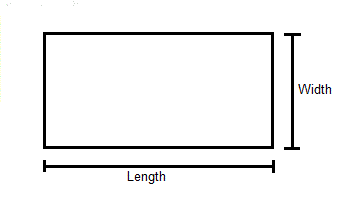Length:
Width:
Rectangular Area:

## Triangular Field Area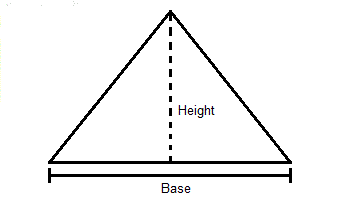Base:
Height:
Triangular Area:

## Circular Field Area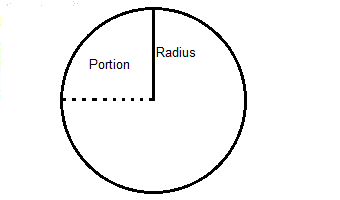Circle Portion:
Circular Area:

## Trapezoidal Field Area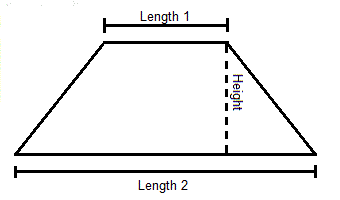Length 1:
Length 2:
Width (Height in the graphic):
Trapezoidal Area:

## The Equations

These Calculators use the following formulas to determine the field area.

#### Rectangular Field AreaWhere: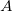= Area of the field (sq. ft)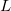= Length of the field (ft)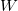= Width of the field (ft)

#### Triangular Field AreaWhere:= Area of the field (sq. ft)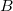= Length of the Base of the field (ft)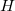= Distance from the base to the top of the field at a 90° from the base (ft)

#### Circular Field Area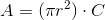Where:= Area of the field (sq. ft)= 3.14159= Radius of the field (ft)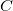= Portion of the circle (as degrees, %, or a decimal)

#### Trapezoidal Field Area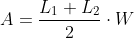Where:= Area of the field (sq. ft)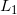= Length of one side of the field (ft)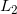= Length of the side Parallel to(ft)= Width of the field, or distance betweenand(ft)

WSU Prosser - IAREC, 24106 N Bunn Rd, Prosser WA 99350-8694, 509-786-2226. Contact Us